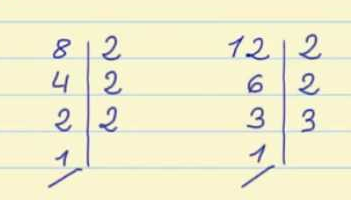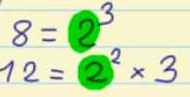Smartick is a fun way to learn math!Dec26

# Greatest Common Factor (GCF)

In this post we are going to learn what the Greatest Common Factor (GCF) is and how to calculate it.

## What is the Greatest Common Factor (GCF)?

It is the biggest number that divides exactly into two or more numbers.

Terms:

• Factor: A factor of a number is a number that divides evenly into the original number, that is to say that the remainder is zero.

Let’s look at an example of this:

### Factors of 20: 1, 2, 4, 5,10, 20

We are going to calculate the factors of 15:

15 / 1 = 15, which means 1 and 15 are factors of 15.

15 / 2 has a remainder of 1, so 2 is not a factor of 15.

15 / 3 = 5, so 3 and 5 are factors of 15.

15 / 4 has a remainder of 3, so 4 is not a factor of 15.

Now we would divide by 5, but since we already know it is a factor, we have finished calculating the factors of 15.

Now we are going to calculate the factors of 20.

20 / 1 = 20, so 1 and 20 are factors of 20.

20 / 2 = 10, so 2 and 10 are factors of 20.

20 / 3 has a remainder of 2, so 3 is not a factor of 20.

20 / 4 = 5, so 4 and 5 are factors of 20.

Now we would divide by 5, but since we already know 5 is a factor of 20, we have finished calculating the factors of 20.

• Common Factor: A number that is simultaneously a factor for two or more numbers, that is to say, is a common factor for those numbers.

If we continue with the previous example, in which we have calculated the factors of 15 and 20, we can now look at the common factors of these two numbers.

In this case, the common factors of 15 and 20 are 1 and 5.

• Greatest Common Factor: The largest number of the common factors.

To continue with the previous example, the Greatest Common Factor of 15 and 20 is 5.

## How do we find the Greatest Common Factor?

Let’s look at different methods for finding the GCF.

• Method 1: We write all the factors of each number, and from these we mark the common factors. The greatest factor marked will be the GCF of those numbers. This method is the one that we have already explained above.
• Method 2: We break down each number into its prime factors. Then, we mark the common prime factors, including repeats. Finally, we multiply the common prime factors.

Let’s look at an example:

### We calculate the GCF of 8 and 12.

Method 1:

• Factors of 8: 1, 2, 4, 8
• Factors of 12: 1, 2, 3, 4, 6, 12
• Common factors of 8 and 12: 1, 2, 4
• Greatest common factor of 8 and 12: 4

Method 2:• Common prime factors, including repeats: 2, 2
• Greatest common factor of 8 and 12: 2 x 2 = 4

We encourage you to practice the exercises in a fun way!

Fun is our brain’s favorite way of learning
Diane Ackerman
Smartick is a fun way to learn math
• 15 fun minutes a day